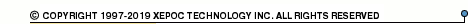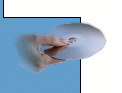More than4 307 195 components listedPWR11 series datasheets. Manufacturer: CANDD.

 PWR1100 1.5 watt unregulated DC/DC converter. Nom.input voltage 5VDC, rated output voltage 5VDC, rated output current 300mA. in 24-pin DIP package. Operational temperature range from -25°C to 85°C. Datasheet*) PWR1101 1.5 watt unregulated DC/DC converter. Nom.input voltage 5VDC, rated output voltage 12VDC, rated output current 125mA. in 24-pin DIP package. Operational temperature range from -25°C to 85°C. Datasheet*) PWR1102 1.5 watt unregulated DC/DC converter. Nom.input voltage 5VDC, rated output voltage 15VDC, rated output current 100mA. in 24-pin DIP package. Operational temperature range from -25°C to 85°C. Datasheet*) PWR1103 1.5 watt unregulated DC/DC converter. Nom.input voltage 5VDC, rated output voltage +-5VDC, rated output current +-150mA. in 24-pin DIP package. Operational temperature range from -25°C to 85°C. Datasheet*) PWR1104 1.5 watt unregulated DC/DC converter. Nom.input voltage 5VDC, rated output voltage +-12VDC, rated output current +-63mA. in 24-pin DIP package. Operational temperature range from -25°C to 85°C. Datasheet*) PWR1105 1.5 watt unregulated DC/DC converter. Nom.input voltage 5VDC, rated output voltage +-15VDC, rated output current +-50mA. in 24-pin DIP package. Operational temperature range from -25°C to 85°C. Datasheet*) PWR1106 1.5 watt unregulated DC/DC converter. Nom.input voltage 12VDC, rated output voltage 5VDC, rated output current 300mA. in 24-pin DIP package. Operational temperature range from -25°C to 85°C. Datasheet*) PWR1107 1.5 watt unregulated DC/DC converter. Nom.input voltage 12VDC, rated output voltage 12VDC, rated output current 125mA. in 24-pin DIP package. Operational temperature range from -25°C to 85°C. Datasheet*) PWR1108 1.5 watt unregulated DC/DC converter. Nom.input voltage 12VDC, rated output voltage 15VDC, rated output current 100mA. in 24-pin DIP package. Operational temperature range from -25°C to 85°C. Datasheet*) PWR1109 1.5 watt unregulated DC/DC converter. Nom.input voltage 12VDC, rated output voltage +-5VDC, rated output current +-150mA. in 24-pin DIP package. Operational temperature range from -25°C to 85°C. Datasheet*) PWR1110 1.5 watt unregulated DC/DC converter. Nom.input voltage 12VDC, rated output voltage +-12VDC, rated output current +-63mA. in 24-pin DIP package. Operational temperature range from -25°C to 85°C. Datasheet*) PWR1111 1.5 watt unregulated DC/DC converter. Nom.input voltage 12VDC, rated output voltage +-15VDC, rated output current +-50mA. in 24-pin DIP package. Operational temperature range from -25°C to 85°C. Datasheet*) PWR1112 1.5 watt unregulated DC/DC converter. Nom.input voltage 15VDC, rated output voltage 5VDC, rated output current 300mA. in 24-pin DIP package. Operational temperature range from -25°C to 85°C. Datasheet*) PWR1113 1.5 watt unregulated DC/DC converter. Nom.input voltage 15VDC, rated output voltage 12VDC, rated output current 125mA. in 24-pin DIP package. Operational temperature range from -25°C to 85°C. Datasheet*) PWR1114 1.5 watt unregulated DC/DC converter. Nom.input voltage 15VDC, rated output voltage 15VDC, rated output current 100mA. in 24-pin DIP package. Operational temperature range from -25°C to 85°C. Datasheet*) PWR1115 1.5 watt unregulated DC/DC converter. Nom.input voltage 15VDC, rated output voltage +-5VDC, rated output current +-150mA. in 24-pin DIP package. Operational temperature range from -25°C to 85°C. Datasheet*) PWR1116 1.5 watt unregulated DC/DC converter. Nom.input voltage 15VDC, rated output voltage +-12VDC, rated output current +-63mA. in 24-pin DIP package. Operational temperature range from -25°C to 85°C. Datasheet*) PWR1117 1.5 watt unregulated DC/DC converter. Nom.input voltage 15VDC, rated output voltage +-15VDC, rated output current +-50mA. in 24-pin DIP package. Operational temperature range from -25°C to 85°C. Datasheet*) PWR1118 1.5 watt unregulated DC/DC converter. Nom.input voltage 24VDC, rated output voltage 5VDC, rated output current 300mA. in 24-pin DIP package. Operational temperature range from -25°C to 85°C. Datasheet*) PWR1119 1.5 watt unregulated DC/DC converter. Nom.input voltage 24VDC, rated output voltage 12VDC, rated output current 125mA. in 24-pin DIP package. Operational temperature range from -25°C to 85°C. Datasheet*) PWR1120 1.5 watt unregulated DC/DC converter. Nom.input voltage 24VDC, rated output voltage 15VDC, rated output current 100mA. in 24-pin DIP package. Operational temperature range from -25°C to 85°C. Datasheet*) PWR1121 1.5 watt unregulated DC/DC converter. Nom.input voltage 24VDC, rated output voltage +-5VDC, rated output current +-150mA. in 24-pin DIP package. Operational temperature range from -25°C to 85°C. Datasheet*) PWR1122 1.5 watt unregulated DC/DC converter. Nom.input voltage 24VDC, rated output voltage +-12VDC, rated output current +-63mA. in 24-pin DIP package. Operational temperature range from -25°C to 85°C. Datasheet*) PWR1123 1.5 watt unregulated DC/DC converter. Nom.input voltage 24VDC, rated output voltage +-15VDC, rated output current +-50mA. in 24-pin DIP package. Operational temperature range from -25°C to 85°C. Datasheet*) PWR1140 1.5 watt unregulated DC/DC converter. Nom.input voltage 5VDC, rated output voltage 9VDC, rated output current 167mA. in 24-pin DIP package. Operational temperature range from -25°C to 85°C. Datasheet*) PWR1141 1.5 watt unregulated DC/DC converter. Nom.input voltage 12VDC, rated output voltage 9VDC, rated output current 167mA. in 24-pin DIP package. Operational temperature range from -25°C to 85°C. Datasheet*) PWR1142 1.5 watt unregulated DC/DC converter. Nom.input voltage 15VDC, rated output voltage 9VDC, rated output current 167mA. in 24-pin DIP package. Operational temperature range from -25°C to 85°C. Datasheet*) PWR11XX 1.5 WATT UNREGULATED DUAL-IN-LINE DC/DC CONVERTER Datasheet*)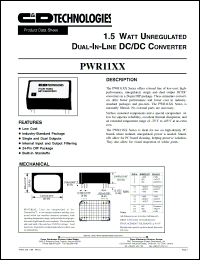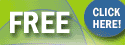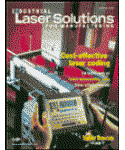Industrial Laser Solutions for Manufacturing Magazine.Subscribe now for FREE !